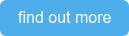<img src="https://trc.taboola.com/1278851/log/3/unip?en=page_view" width="0" height="0" style="display:none">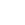0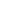7 min

This blog will cover an information regarding moving the data between cubes using Rules and Feeders. Questions to be asked before we get started with the topic... Why do we need to move the data to another cube which is having similar dimension structure….?? Can’t we just modify the existing cube….?? Answers “we can modify the cube” and “there is no need to create a duplicate cube “, although ...This blog will cover an information regarding moving the data between cubes using Rules and Feeders.

Questions to be asked before we get started with the topic...

Why do we need to move the data to another cube which is having similar dimension structure….??

Can’t we just modify the existing cube….??

Answers “we can modify the cube” and “there is no need to create a duplicate cube “, although there can be a few exceptions like dependent cube(s) which might have been used to create report which sometimes a client may request not to modify.

Ideally the data can be moved between cubes using TI process by taking Data Source as “Cube View” but we do have the option to push the data using RULES and FEEDERS.

There are 3 cases to consider while copying the data:

1. Copy data from one cube to another having same number of dimensions
2. Copy data from one cube to another having less number of dimensions in destination cube.
3. Copy data from one cube to another having more number of dimensions in destination cube.

Scenario 1: Copy data from cube1 to cube1_1 having same number of dimension

There is a Cube called “Cube1”, which has 4 dimensions with value.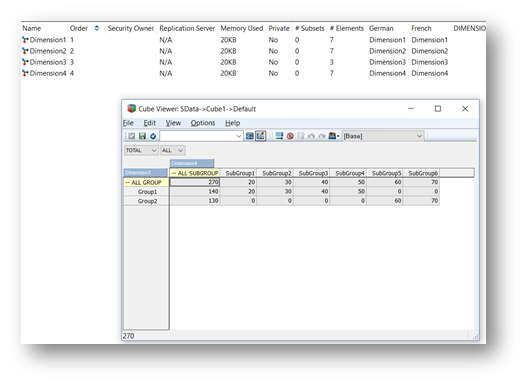Fig 1.1  Cube1 structure

As per the requirement, “Cube1” data has to move to “Cube1_1” (both cubes have similar structure).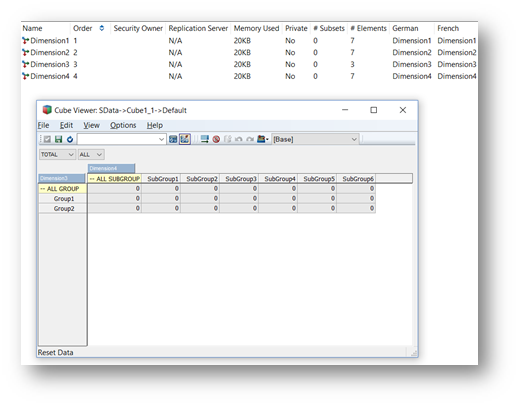Fig 1.2 Cube1_1 structure

Now in moving the data using RULES and FEEDER to Cube1_1 (Destination Cube) from Cube1 (Source Cube):

RULES:  Rules need to be written in the Destination Cube (Cube1_1).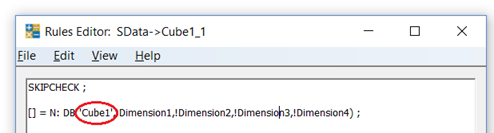Fig 1.3 Rules for Cube1_1• In DB function, the cube name should be the “Source Cube (Cube1)” – where the data is present
• It is not mandatory to have the same Dimension name in both cubes, but Element names should be same

FEEDERS: What data need to be written into the destination cube (Cube1_1) must be written in the Source cube (Cube1).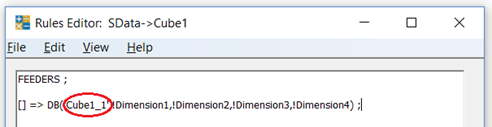Fig 1.4 Feeders for Cube1_1 (written in Cube1)• In DB function, the cube name should be the “Destination Cube (Cube1_1)” – where the data need to be fed

After this, you just need to open the Cube1_1 and press on recalculate then will have the value.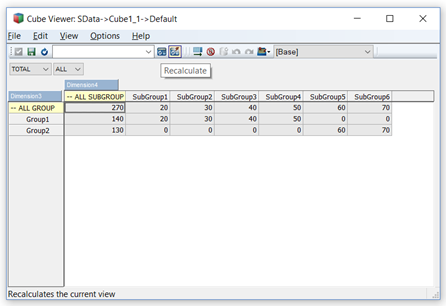Scenario 2: Copy data from cube1 to cube1_2 having less number of dimension in destination cube.

There is a cube called “Cube1”, which has 4 dimensions with value.Fig 2.1  Cube1 structure

As per the requirement, “Cube1” data has to move to “Cube1_2” (less number of dimension in destination cube).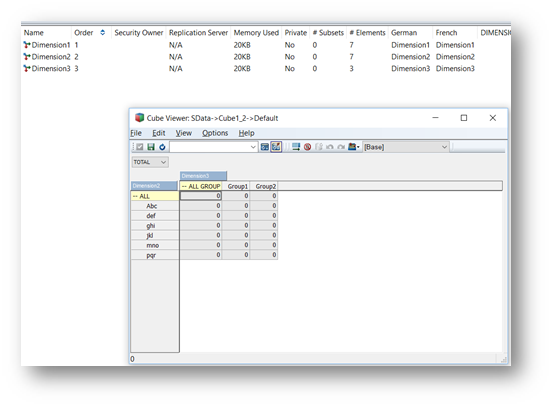\

Fig 2.2 Cube1_2 structure

Now moving the data using RULES and FEEDER to Cube1_2 (Destination Cube) from Cube1 (Source Cube):

RULES:  Rules need to be written in the Destination Cube (Cube1_2).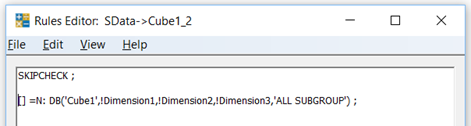Fig 2.3 Rules for Cube1_2• In DB function, the cube name should be the “Source Cube (Cube1)” – where the data is present
• It is not mandatory to have the same Dimension name in both cubes, but Element names should be same

FEEDERS: What data need to be written into the destination cube (Cube1_2) must be written in the Source cube (Cube1).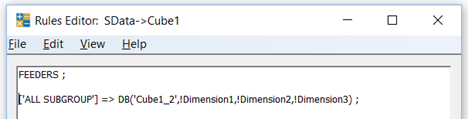Fig 2.4 Feeders for Cube1_2 (written in Cube1)• In DB function, the cube name should be the “Destination Cube (Cube1_2)” – where the data need to be fed

After this, just need to open the Cube1_2 and press on recalculate then will have the value.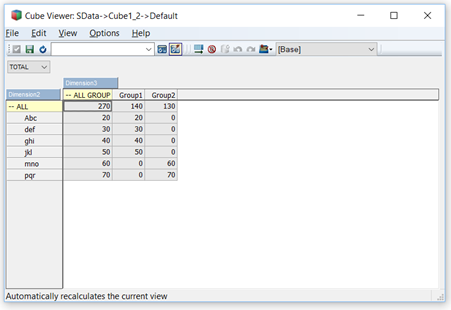Scenario 3: Copy data from one cube to another having more dimensions in the destination cube

There is a cube called “Cube1”, which has 4 dimensions with value.Fig 3.1  Cube1 structure

As per the requirement, “Cube1” data has to move to “Cube1_3” (more number of dimension in destination cube)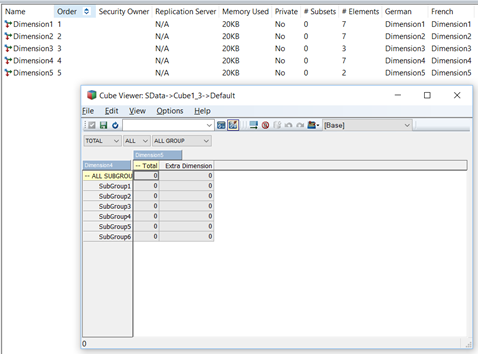Fig 3.2 Cube1_3 structure

Now moving the data using RULES and FEEDER to Cube1_3 (Destination Cube) from Cube1 (Source Cube):

RULES:  Rules need to be written in the Destination Cube (Cube1_3).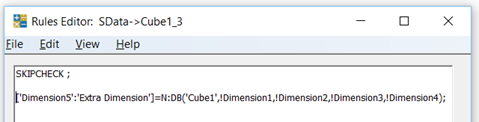Fig 3.3 Rules for Cube1_3• In DB function, the cube name should be the “Source Cube (Cube1)” – where the data is present
• It is not mandatory to have the same Dimension name in both cubes, but Element names should be same

FEEDERS: What data need to be written into the destination cube (Cube1_3) must be written in the Source cube (Cube1).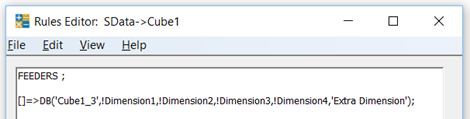Fig 3.4 Feeders for Cube1_3 (written in Cube1)• In DB function, the cube name should be the “Destination Cube (Cube1_3)” – where the data need to be fed

After this, just need to open the Cube1_3 and press on recalculate then will have the value.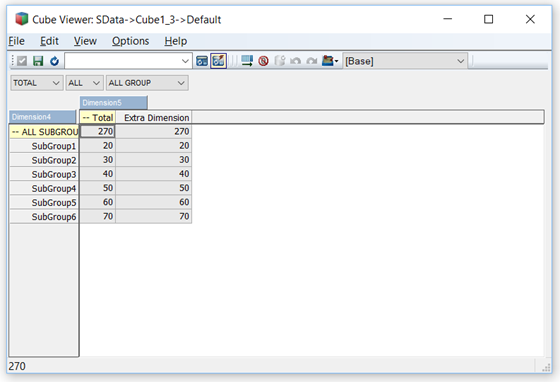## Try out our TM1 \$99 training Join our TM1 training session from the comfort of your home